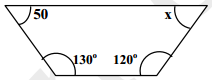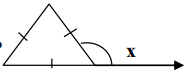# MCQ Questions for Class 8 Maths Chapter 4 Practical Geometry with Answers

Free PDF Download of CBSE Maths Multiple Choice Questions for Class8 with Answers Chapter 4 Practical Geometry. Maths MCQs for Class 8 Chapter Wise with Answers PDF Download was Prepared Based on Latest Exam Pattern. Students can solve NCERT Class 8 Maths Practical Geometry MCQs Pdf with Answers to know their preparation level.

## Practical Geometry Class 8 Maths MCQs Pdf

1. To construct a square, we need to know:
a) All the interior angles
b) All the side lengths
c) Only one interior angle
d) Only one side length

Explanation: A square has all its sides equal and all the interior angles measure 90 degrees. Hence, if the length of one side is known, then we can construct a square easily.

2. To construct a parallelogram we need to know:
a) Length of its parallel sides
b) Measure of interior angles
c) Two adjacent sides and one angle
d) Two adjacent sides and two angles

Explanation: Parallelogram has its parallel sides equal. Also, if one angle is known to us, then we can determine the other angle since the two angles are supplementary.

3. To construct a rectangle, we need to know:
a) All the interior angles
b) All the Sides
d) Only one angle measure

Explanation: A rectangle has its parallel sides equal and all the interior angles measure 90 degrees. Hence, if the length and breadth rectangle is known, then we can construct it easily.

4. If two diagonals are given, then we can construct a:
a) Rhombus
b) Rectangle
c) Kite
d) Parallelogram

Explanation: The two diagonals of a rhombus bisect each other at 90 degrees.

5. What is the appropriate condition to construct a quadrilateral?
a) When four sides and one diagonal are given
b) When three sides and one diagonal are given
c) When two sides and one diagonal are given
d) None of the above

6. If two diagonals and three sides are given, then:
a) A quadrilateral cannot be constructed
b) A quadrilateral can be constructed
c) Insufficient information
d) Any polygon can be constructed

7. To construct a quadrilateral, we need to know two adjacent side and _____ angles.
a) One
b) Two
c) Three
d) All four angles

8. To construct a quadrilateral, we need to know two diagonals and _____ sides.
a) One
b) Two
c) Three
d) All four sides

9. To construct a quadrilateral, we need to know three sides and _____ included angles.
a) One
b) Two
c) Three
d) All four angles

10. How many measurements are required to construct a quadrilateral, uniquely?
a) Four
b) Five
c) Six
d) Three

11. A simple closed curve made up of only ______________ is called a polygon .
(a)curves
(b) line segments
(c) lines
(d) closed curves

12. A polygon with minimum number of sides is
(a) Pentagon
(b) Square
(c) triangle
(d) angle

13. Polygons that have no portions of their diagonals in their exteriors are called
(a) Squares
(b) triangles
(c) convex
(d) concave

14. Polygons that have any portions of their diagonals in their exteriors are called
(a) Squares
(b) triangles
(c) convex
(d) concave

15. All the sides of a regular polygon are ______________.
(a) Parallel
(b) equal in length
(c) not parallel
(d) not equal

16. All the angles of a regular polygon are of ______________.
(a) 90°
(b) 60°
(c) equal measure
(d) equal length

17. Sum of all interior angles of a polygon with (n) sides is given by
(a) (n – 2 ) × 180°
(b) n – 2 × 180°
(c) (n + 2 ) × 180°
(d) n + 2 × 180°

18. Maximum number of right angles in a right angled triangle are
(a) 2
(b) 1
(c) 3
(d) 0

19. Sum of all interior angles of a parallelogram is
(a) 180°
(b) 360°
(c) 540°
(d) 240°

20. The angle sum of all interior angles of a convex polygon of sides 7 is
(a) 180°
(b) 540°
(c) 630°
(d) 900°

21. Each exterior angle of a regular hexagon is of measure
(a) 120°
(b) 80°
(c)100°
(d) 60°

22. The number of sides in a regular polygon is 15 , then measure of each exterior angle is
(a) 24°
(b) 36°
(c)20°
(d)18°

23. The measure of each interior angle of a regular polygon is 140o, then number of sides that regular polygon has ______________.
(a) 15
(b) 12
(c) 9
(d) 10

24. Which of the following polygons is convex polygon ?25. Which of the following is concave polygon?26. The value of (x) in the following figure is(a) 120°
(b) 80°
(c) 100°
(d) 60°

27. A quadrilateral which has 2 pairs of equal adjacent sides but unequal opposite sides is called ______________.
(a) parallelogram
(b) rhombus
(c) kite
(d) square

28. The value of x in the following figure is(a) 100°
(b) 90°
(c) 108°
(d) 120°

29. The value of x in the following figure is(a) 120°
(b) 180°
(c) 60°
(d) 100°

30. A parallelogram each of whose angles measures 90o is ______________.
(a) rectangle
(b) rhombus
(c) kite
(d) trapezium

31. A parallelogram whose all sides are equal is called
(a) square
(b) rhombus
(c) rectangle
(d) trapezium

32. the diagonals of a rhombus bisect each other at ______________ angles.
(a) acute
(b) right
(c) obtuse
(d) reflex

33. Diagonals of a rectangle:
(a) equal to each other
(b) not equal
(c) one is double of the other
(d) none of these

34. The diagonals of a square bisect each other at ______________ angle.
(a) acute
(b) right
(c) obtuse
(d) reflex

35. the value of x in the following figure is:
(a) 60°
(b) 70°
(c) 180°
(d) 90°

36. Minimum possible interior angle in a regular polygon is ______________.
(a) 70°
(b) 60°
(c) 90°
(d) 120°

37. Maximum possible exterior angle in a regular polygon is ______________.
(a) 70°
(b) 60°
(c) 90°
(d) 120°

38. How many sides does a heptagon have ?
(a) 2
(b) 4
(c) 7
(d) 5

39. Name the closed figure with 4 sides ?
(a) Hexagon
(b) Triangle
(c) Pentagon

40. How many diagonals does a regular Hexagon has ?
(a) 2
(b) 9
(c) 3
(d) 5

41. What is the number of sides in Hexagon ?
(a) 4
(b) 7
(c) 6
(d) 5

42. What is the sum of the measures of angles of a convex quadrilaterals?
(a) 180°
(b) 90°
(c) 360°
(d) 45°

43. If the three angles of a quadrilateral are 120o , 130o, 10o then what is the fourth angle ?
(a) 30°
(b) 100°
(c) 40°
(d) 90°

44. The opposite angles of a parallelogram are ______________ .
(a) Unequal
(b) equal
(c) complementary
(d) supplementary

45. The perimeter of parallelogram PQRS is:(a) 12 cm
(b) 7 cm
(c) 38 cm
(d) 19 cm

46. The diagonals of a square are ______________ each other
(a) equal to
(b) unequal to
(c) perpendicular bisectors of
(d) none of these

47. A parallelogram with sides of equal length is called______________.
(a) trapezium
(b) square
(c) rectangle
(d) rhombus

48. How many measurements can determine a quadrilateral uniquely?
(a) 2
(b) 3
(c) 4
(d) 5

49. Diagonals of a parallelogram _______________ each other.
(a) bisect
(b)equal to
(c)perpendicular to
(d)none of these

50.How many sides does decagon has?
(a) 8
(b) 10
(c) 6
(d)